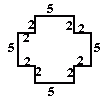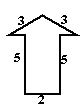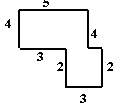Name: ___________________Date:___________________

 Email us to get an instant 20% discount on highly effective K-12 Math & English kwizNET Programs!

### Grade 7 - Mathematics9.39 Finding Perimeter of Polygons

 Q 1: Find the perimeter of the figure.Answer: Q 2: Find the perimeter of regular octagon with length 2 cms.Answer: Q 3: Find the perimeter of regular hexagon with length 3 cms.Answer: Q 4: Find the perimeter of the quadrilateral.Answer: Q 5: Find the perimeter of the figure.Answer: Q 6: Find the perimeter of regular pentagon of length 4 cms.Answer: Q 7: Find the perimeter of the figure.Answer: Q 8: Find the perimeter of the triangle.Answer: Question 9: This question is available to subscribers only! Question 10: This question is available to subscribers only!